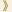HomeShaw Laureates2015Mathematical SciencesEssay

The theory of numbers is one of the oldest branches of mathematics, going back more than two thousand years in China, Greece, and India. It is concerned with the study of whole numbers, prime numbers, and polynomial equations involving them. The third of these goes by the name Diophantine equations after the Alexandrian/Greek mathematician Diophantus. Gauss, who laid many of the foundations of modern number theory, called it the “Queen of Mathematics”. At the time, and for many years after, it was considered as very much on the theoretical and pure side of mathematics. However, in our modern digital/discrete world the deeper truths and techniques that have been developed to study whole numbers play an increasingly significant role in applications.

Many of the central problems in the theory of numbers are elementary and easy to state; but the experience of generations of mathematicians shows that they can be extraordinarily difficult to resolve. Success, when it is achieved, often relies on sophisticated tools from many fields of mathematics. This is no coincidence, since aspects of these fields were introduced and developed in efforts to resolve classical problems in number theory.

Faltings and Iwaniec have developed many of the most powerful modern tools in algebra, analysis, algebraic and arithmetic geometry, automorphic forms, and the theory of zeta functions. They and others have used these tools to resolve longstanding problems in number theory.

Gerd Faltings

A polynomial equation of degree n in one variable with coefficients which are rational numbers has just n complex numbers as solutions. Such an equation has a symmetry group, its Galois group, that describes how these complex solutions are related to each other.

A polynomial equation in two variables with rational coefficients has infinitely many complex solutions, which form an algebraic curve. In most cases (that is, when the curve has genus 2 or more) such a Diophantine equation has only finitely many solutions whose coordinates are rational numbers. This statement was for over sixty years a famous conjecture of Mordell, until Faltings finally proved it. His unexpected proof provided fundamental new tools in Arakelov and arithmetic geometry, as well as a proof of another fundamental finiteness theorem — the Shaferavich and Tate Conjecture — concerning polynomial equations in many variables. Later, developing a quite different method of Vojta, Faltings established a far-reaching higher dimensional finiteness theorem for rational solutions to systems of equations on Abelian varieties (the Lang conjectures). In order to study rational solutions of polynomial equations by geometry, one needs arithmetic versions of the tools of complex geometry. One such tool is Hodge theory. Faltings' foundational contributions to Hodge theory over the p-adic numbers, as well as his introduction of other related novel and powerful techniques, are at the core of some of the recent advances connecting Galois groups (from polynomial equations in one or more variables) and the modern theory of automorphic forms (a vast generalization of the theory of periodic functions). The recent striking work of Peter Scholze concerning Galois representations is a good example of the power of these techniques.

Henryk Iwaniec

Iwaniec’s work concerns the analytic side of Diophantine analysis, where the goal is usually to prove that equations do have integral or prime solutions, and ideally to estimate how many there are up to a given size.

One of the oldest techniques for finding primes is sieve theory, originating in Erastosthenes’ description of how to list the prime numbers. Iwaniec's foundational works and breakthroughs in sieve theory and its applications form a large part of this active area of mathematics. His proof (with John Friedlander) that there are infinitely many primes of the form X2+Y4 is one of the most striking results about prime numbers known; the techniques introduced to prove it are the basis of many further works. The theory of Riemann's zeta function — and more generally of L-functions associated with automorphic forms — plays a central role in the study of prime numbers and Diophantine equations. Iwaniec invented many of the powerful techniques for studying L-functions of automorphic forms, which are used widely today. Specifically, his techniques to estimate the Fourier coefficients of modular forms of half-integral weight and for estimating L-functions on their critical lines (the latter joint with William Duke and John Friedlander) have led to the solution of a number of longstanding problems in number theory, including one of Hilbert's problems: that quadratic equations in integers (in three or more variables) can always be solved unless there is an “obvious” reason that they cannot.

In a series of papers remarkable both in terms of their concept and novel techniques, Iwaniec together with different authors (Étienne Fouvry and then Enrico Bombieri and John Friedlander), established results about the distribution of primes in arithmetic progressions which go beyond the notorious Riemann hypothesis. This opened the door to some potentially very striking applications. Yitang Zhang’s much celebrated recent result on bounded gaps between primes relies heavily on the works of Iwaniec et al. Iwaniec’s work mentioned above, together with his many other technically brilliant works, have a central position in modern analytic number theory.

24 September 2015   Hong Kong